# Absolute Value

The absolute value of a number, according to the most accurate and most useful definition, is that number’s distance from zero on the number line.  For example, if the absolute value of x is 5, then the distance between x and 0 is 5. This represents two possibilities, as we can see on this number line. Namely, x is either 5 or -5: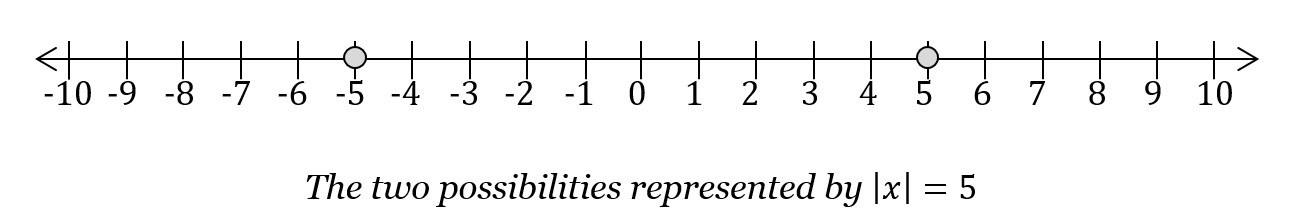“Distance on the number line” is probably not how absolute value was first described to you. Absolute value notation is usually taught as, “If you see a negative sign in front of the number, change it to a plus sign. If you see a plus sign, leave it alone.” In other words, absolute value makes numbers positive. The absolute value of -17 is +17. The absolute value of +36 is +36. This definition is less accurate and less useful, but it’s still worth knowing.

The more formal definition of absolute value, which is equivalent to both of the ideas above, is the following: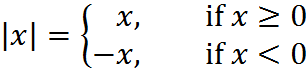If the original number is zero or positive, then the absolute value of the number is simply the original number. If the number is negative, then the absolute value is the original number with the negative sign stripped away. For example: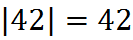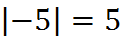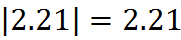Notice that an equation with an absolute value represents two possibilities. This fact is important on some GMAT questions, in which you have two cases to analyze – one for each side on the number line.

## Exercises

1. Calculate the absolute value of the following numbers:

a. -5

b. 9

c. -3.8

d. -139,462

e. 5/8

2. What is the absolute value of zero? Explain.

3. Draw a graph of abs(x) from -3 to +3. Can abs(x) ever be less than zero? How can you see that from your graph?

1. a. 5; b. 9; c. 3.8; d. 139,462; e. 5/8

2. Zero, because zero is exactly zero away from zero on the number line.

3. Graph of the absolute value of x: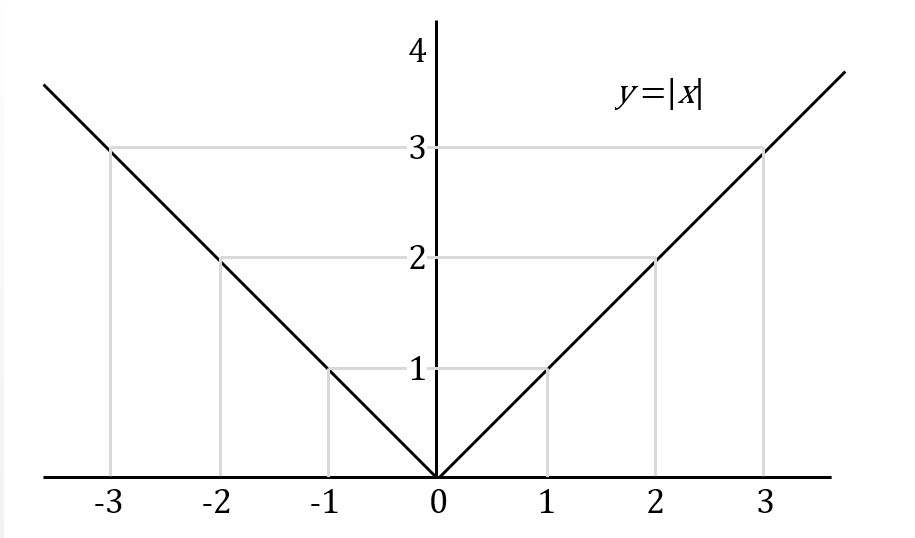The graph shows the absolute value of a number can never be less than zero, because the graph has a minimum value of 0 and is never negative, never below the x-axis.

## Practice Questions

Absolute Value on Number Line:
http://www.gmatfree.com/absolute-value-on-number-line

Absolute Value Inequality:
http://www.gmatfree.com/absolute-value-inequality

Possible Absolute Values:
http://www.gmatfree.com/possible-absolute-values

Welcome! You are encouraged to register with the site and login (for free). When you register, you support the site and your question history is saved.